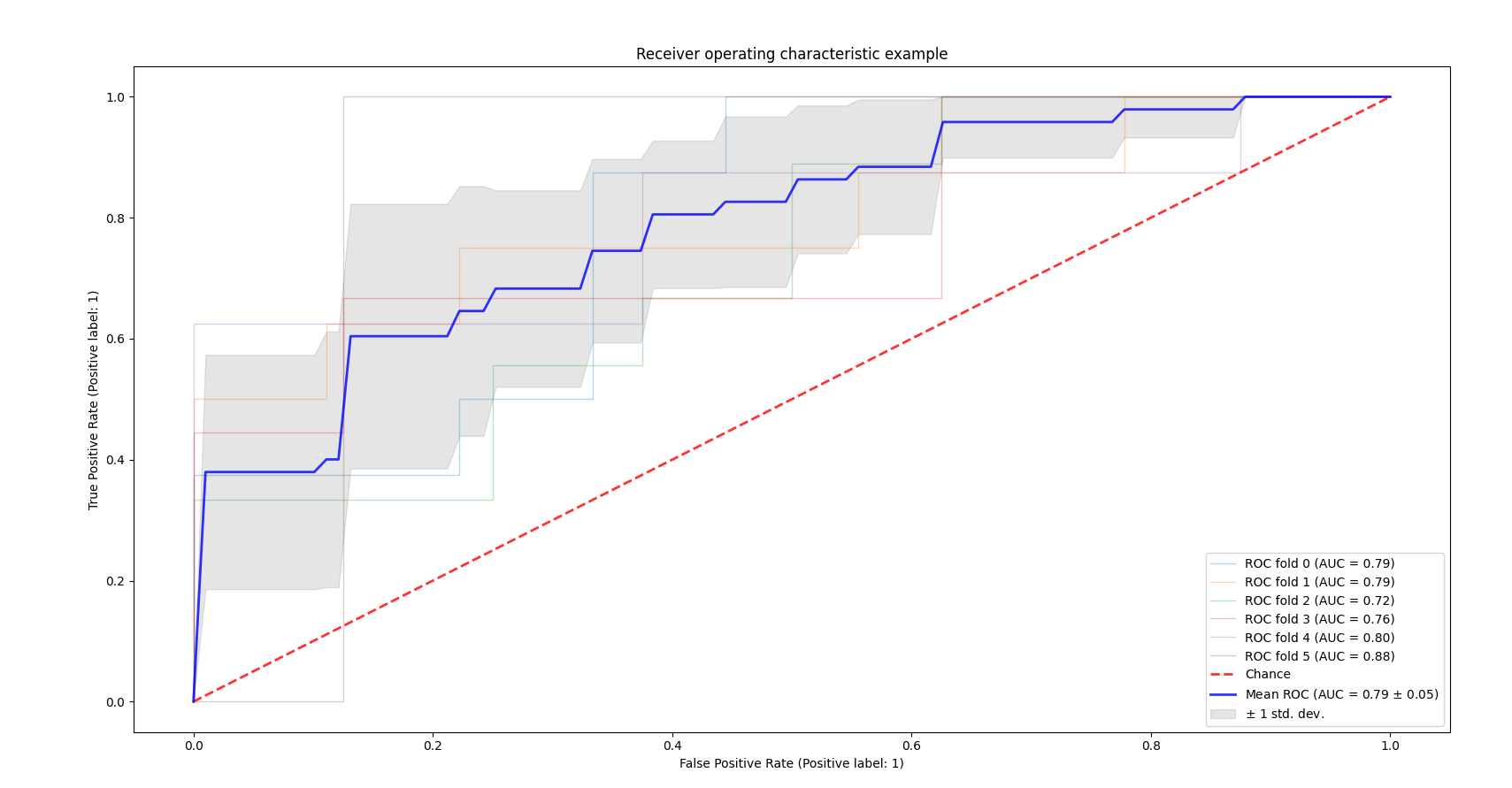• 交叉验证曲线
千次阅读
2019-05-29 17:13:10

scikit-learn中有非常多的曲线，对于调参非常有用处，我经常用到的曲线是学习曲线，验证曲线以及交叉验证曲线。

使用这些曲线的代码格式非常固定：

返回值 = 曲线名字（模型对象，X, Y, 交叉验证数量，评分标准.....）

它们只是返回值不同以及其中设置的参数略有不同。

### 学习曲线：（learning curve）

这条曲线的主要作用是观察数据集的大小对于模型性能的影响。

使用代码如下：

train_sizes, train_scores, valid_scores = learning_curve(
SVC(kernel='linear'), X, y, train_sizes=[50, 80, 110], cv=5，scoring='r2')

其中train_sizes=[50, 80, 110]的意思是选择50个、80个、110个样本数据，还可以[0.1,0.2,1.0]这样设置，这就是按照比例来分割数据的意思。

### 验证曲线：（validation curve）

这条曲线的用处主要是观察超参数对模型带来的影响，为模型选择合适的超参数。

使用代码如下：

sklearn.learning_curve.validation_curve(estimatorXyparam_nameparam_rangecv=Nonescoring=Nonen_jobs=1pre_dispatch='all'verbose=0)

train_scores, valid_scores = validation_curve(Ridge(), X, y, "超参数的名称",                                               np.logspace(-7, 3, 3))

### 交叉验证曲线 （cross_val_score)

from sklearn.cross_validation import cross_val_score

scores = cross_val_score(knn, X, y, cv=10, scoring='accuracy')

以上是调节模型参数的三种曲线，使用非常方便。sklearn python 机器学习
更多相关内容
• 交叉验证的ROC曲线前言一、程序如下二、效果图 前言 ROC曲线通常在Y轴上具有真正率（true positive rate），在X轴上具有假正率(false positive rate)。这意味着该图的左上角是“理想”点-误报率为零，而正误报率...

# 前言

ROC曲线通常在Y轴上具有真正率（true positive rate），在X轴上具有假正率(false positive
rate)。这意味着该图的左上角是“理想”点-误报率为零，而正误报率为1。这不是很现实，但这确实意味着，通常来说，曲线下的区域（AUC面积）越大越好。

ROC曲线的“陡峭程度”也很重要，因为理想的是最大程度地提高真正率，同时最小化假正率。

此示例显示了通过K折交叉验证创建的不同数据集的ROC曲线。取所有这些曲线，可以计算出曲线下的平均面积，并在训练集分为不同子集时查看曲线的方差。这大致显示了分类数据的输出如何受到训练数据变化的影响，以及由K折交叉验证产生的拆分之间的差异如何。

# 一、程序如下

import numpy as np
import matplotlib.pyplot as plt

from sklearn import svm, datasets
from sklearn.metrics import auc
from sklearn.metrics import plot_roc_curve
from sklearn.model_selection import StratifiedKFold

# #############################################################################
# 获取数据

# 导入待处理的数据
X = iris.data
y = iris.target
X, y = X[y != 2], y[y != 2]
n_samples, n_features = X.shape

# 增加一些噪音特征
random_state = np.random.RandomState(0)
X = np.c_[X, random_state.randn(n_samples, 200 * n_features)]

# #############################################################################
# 分类，并使用ROC曲线分析结果

# 使用交叉验证运行分类器并绘制ROC曲线
cv = StratifiedKFold(n_splits=6)
classifier = svm.SVC(kernel='linear', probability=True,
random_state=random_state)

tprs = []
aucs = []
mean_fpr = np.linspace(0, 1, 100)

fig, ax = plt.subplots()
for i, (train, test) in enumerate(cv.split(X, y)):
classifier.fit(X[train], y[train])
viz = plot_roc_curve(classifier, X[test], y[test],
name='ROC fold {}'.format(i),
alpha=0.3, lw=1, ax=ax)
interp_tpr = np.interp(mean_fpr, viz.fpr, viz.tpr)
interp_tpr = 0.0
tprs.append(interp_tpr)
aucs.append(viz.roc_auc)

ax.plot([0, 1], [0, 1], linestyle='--', lw=2, color='r',
label='Chance', alpha=.8)

mean_tpr = np.mean(tprs, axis=0)
mean_tpr[-1] = 1.0
mean_auc = auc(mean_fpr, mean_tpr)
std_auc = np.std(aucs)
ax.plot(mean_fpr, mean_tpr, color='b',
label=r'Mean ROC (AUC = %0.2f $\pm$ %0.2f)' % (mean_auc, std_auc),
lw=2, alpha=.8)

std_tpr = np.std(tprs, axis=0)
tprs_upper = np.minimum(mean_tpr + std_tpr, 1)
tprs_lower = np.maximum(mean_tpr - std_tpr, 0)
ax.fill_between(mean_fpr, tprs_lower, tprs_upper, color='grey', alpha=.2,
label=r'$\pm$ 1 std. dev.')

ax.set(xlim=[-0.05, 1.05], ylim=[-0.05, 1.05],
title="Receiver operating characteristic example")
ax.legend(loc="lower right")
plt.show()



# 二、效果图展开全文python 人工智能 机器学习
• 我们可以通过学习曲线来检测模型是欠拟合，过拟合还是刚刚好。
• 一、交叉验证 1. 数据集划分： sklearn.cross_validation.KFold(n, n_folds=3, shuffle=False, random_state=None)  参数说明：  n: 要参与到交叉验证中来的元素个数，一般是全选（如下例中5）  n_folds = 3:...

一、交叉验证

1. 数据集划分：

sklearn.cross_validation.KFold(n,n_folds=3,shuffle=False,random_state=None)

参数说明：

n: 要参与到交叉验证中来的元素个数，一般是全选（如下例中5）

n_folds = 3: 要分成几堆，也就是K值，默认3，视机器性能进行选择，可选5、7、10等

shuffle = False: 是否打乱原有顺序呢

代码示例：输出结果：

X_train: [[ 7 8]，[ 9 10]] y_train: [4 5]
X_test: [[1 2]，[3 4]，[5 6]] ytest: [1 2 3]
X_train: [[1 2]，[3 4]，[5 6]] y_train: [1 2 3]
X_test: [[ 7 8]，[ 9 10]] ytest: [4 5]

也可以使用train_test_split，这里的参数很容易理解。

代码示例及结果：2. 交叉验证得分：

二、学习曲线

用于判断模型是否过拟合，当模型在训练集上得分很高，但是在交叉验证集上得分很低时，模型过拟合

若模型未过拟合，可以考虑继续挖掘更多特征

import numpy as np
import matplotlib.pyplot as plt
from sklearn.learning_curve import learning_curve

# 用sklearn的learning_curve得到training_score和cv_score，使用matplotlib画出learning curve
def plot_learning_curve(estimator, title, X, y, ylim=None, cv=None, n_jobs=1,
train_sizes=np.linspace(.05, 1., 20), verbose=0, plot=True):
"""
画出data在某模型上的learning curve.
参数解释
----------
estimator : 你用的分类器。
title : 表格的标题。
X : 输入的feature，numpy类型
y : 输入的target vector
ylim : tuple格式的(ymin, ymax), 设定图像中纵坐标的最低点和最高点
cv : 做cross-validation的时候，数据分成的份数，其中一份作为cv集，其余n-1份作为training(默认为3份)
n_jobs : 并行的的任务数(默认1)
"""
train_sizes, train_scores, test_scores = learning_curve(
estimator, X, y, cv=cv, n_jobs=n_jobs, train_sizes=train_sizes, verbose=verbose)

train_scores_mean = np.mean(train_scores, axis=1)
train_scores_std = np.std(train_scores, axis=1)
test_scores_mean = np.mean(test_scores, axis=1)
test_scores_std = np.std(test_scores, axis=1)

if plot:
plt.figure()
plt.title(title)
if ylim is not None:
plt.ylim(*ylim)
plt.xlabel(u"训练样本数")
plt.ylabel(u"得分")
plt.gca().invert_yaxis()
plt.grid()

plt.fill_between(train_sizes, train_scores_mean - train_scores_std, train_scores_mean + train_scores_std,
alpha=0.1, color="b")
plt.fill_between(train_sizes, test_scores_mean - test_scores_std, test_scores_mean + test_scores_std,
alpha=0.1, color="r")
plt.plot(train_sizes, train_scores_mean, 'o-', color="b", label=u"训练集上得分")
plt.plot(train_sizes, test_scores_mean, 'o-', color="r", label=u"交叉验证集上得分")

plt.legend(loc="best")

plt.draw()
plt.show()
plt.gca().invert_yaxis()

midpoint = ((train_scores_mean[-1] + train_scores_std[-1]) + (test_scores_mean[-1] - test_scores_std[-1])) / 2
diff = (train_scores_mean[-1] + train_scores_std[-1]) - (test_scores_mean[-1] - test_scores_std[-1])
return midpoint, diff

plot_learning_curve(clf, u"学习曲线", X, y)

展开全文kaggle 机器学习
• 样本数量不一致的交叉验证ROC可视化。包含了交叉验证的值范围。

最近在画交叉验证的ROC曲线。由于我采用的是留一法来做10折交叉，这就导致我每一折的样本数量是不固定的，但我又想画一个平均的校验验证性能曲线，并且把置信区间也画出来，找了很久资料，我画了一个这样的。先看效果图，如下图：中间蓝色的是平均的ROC，周围蓝色的带是所有的交叉验证触及的最大范围。因为交叉验证需要多次抽样，但我用的是深度学习，很难做到抽样，所以就用最大范围代替置信区间。具体做法如下：

from my_utils.utils import *
from sklearn.metrics import auc, plot_roc_curve, roc_curve
import my_utils.file_util as fu
import matplotlib.pyplot as plt
class_type = '1p19q'
#图表的保存路径
save_path = ''
#存放所有交叉验证的预测结果和label的文件路径
record_dir  = ''
#find_file是我自己写的寻找路径下所有best-auc.csv文件的函数，用的时候可以替换成自己的
#返回的是所有csv文件的绝对路径。文件格式是第一和第二列是预测值，第三列label。
#因为我用的是one hot做二分类，所以有两个值
flods_dir = find_file(record_dir, 2,1, suffix='best_auc.csv')
pre_record = []
#获取所有交叉验证的预测值和label
for record_file in flods_dir:
temp_fold_record = []
#因为是二分类，所有只取第一个预测值，第二个不要
temp_fold_record.append([i for i in temp])
#取label值
temp_fold_record.append([i for i in temp])
pre_record.append(temp_fold_record)

roc_record, value, fpr, tpr = [], [], [], []

for record in pre_record:
label = np.array([int(i) for i in record]).squeeze()
pred = np.array([float(i) for i in record]).squeeze()
#计算roc曲线
t_fpr, t_tpr, thre = roc_curve(label, 1-pred)
#计算AUC
auc_score = auc(t_fpr, t_tpr)
fpr.append([float(i) for i in t_fpr])
value.extend([float(i) for i in t_fpr])
tpr.append([float(i) for i in t_tpr])

mean_fpr, min_fpr, max_fpr = [],[],[]
mean_tpr, min_tpr, max_tpr= [],[],[]
#这个是为了对曲线进行插值，因为每个曲线的样本不一样，所以获取到的fpr和tpr也不一样长度，
#所以需要进行插值
#插值的原理是，获取所有fpr的值，然后将每个交叉验证的roc都插值成和fpr的值一样多的长度。
#插值并不会改变每个roc曲线的形状，这个可以放心使用
unique = np.unique(np.array(value).flatten())
#对fpr和tpr进行插值
for l in range(len(fpr)):
tpr[l] = np.interp(unique, fpr[l], tpr[l], 0, 1)
fpr[l] = np.interp(unique, fpr[l], fpr[l], 0, 1)
#将每个交叉验证的ROC都画出来
plt.plot(fpr[l], tpr[l])

fpr = np.array(fpr)
tpr = np.array(tpr)
#求fpr和tpr的极大极小，获取每个实验所能触及的最大指标范围
min_fpr = np.min(fpr, axis=0)
max_fpr = np.max(fpr, axis=0)
min_tpr = np.min(tpr, axis=0)
max_tpr = np.max(tpr, axis=0)
#获取均值，为了得到交叉验证的平均曲线
mean_tpr = np.mean(tpr, axis=0)
mean_fpr = np.mean(fpr, axis=0)
mean_tpr = 0

plt.figure(dpi=300)
plt.title(class_type)
plt.style.use('seaborn')
plt.xlabel('1-Specificity')
plt.ylabel('Sencitivity')
#画平均曲线
p1 = plt.plot(mean_fpr, mean_tpr, marker='.',
linestyle='--',c='b', linewidth=1,
alpha=0.65, label='20X')#markeredgecolor='b',

plt.legend(loc='lower right')
#将极大极小值的范围填充淡蓝色
p2 = plt.fill_between(min_fpr,max_tpr, min_tpr,color='blue', alpha=0.08)
#展示图象
plt.show()



下面附上每个交叉验证的图像来验证范围画的对不对。再对比我们画的可以看到啊，我们画的范围与所有ROC的曲线的范围是吻合的，平均曲线也像模像样。

展开全文python 机器学习 ROC 交叉验证
• Matlab 实现多项式曲线拟合（正弦曲线），交叉验证（十折交叉验证
• 什么是交叉验证 基本思想就是将原始数据（dataset）进行分组，一部分做为训练集来训练模型，另一部分做为测试集来评价模型。 为什么要做交叉验证 交叉验证用于评估模型的预测性能，尤其是训练好的模型在新数据上...交叉验证 k折交叉验证 留一法
• 目录1 交叉验证2 Python\rm{}PythonPython绘制交叉验证AUROC\rm{}AUROCAUROC3 Python\rm{}PythonPython绘制交叉验证AURR\rm{}AURRAURR4 参考文献 1 交叉验证    2 Python\rm{}PythonPython绘制交叉验证AUROC\rm{}...python AUROC 交叉验证
• ## Stata: 交叉验证简介

千次阅读 2019-10-30 00:43:52
作者：贺旭 （中央财经大学） Stata连享会   计量专题 || 精品课程 || 简书推文 || 公众号合集 ...本文介绍交叉验证方法，然后以 kfoldclass 命令和 crossfold 为范例使读者更深入的了解该方法。 ...交叉验证
• 在绘制交叉验证的平均ROC曲线时采用将五折的fpr和tpr求平均以获得最终tpr和fpr，这么做有依据吗？以前前面部分求插值的操作，是在有点看不懂，有大神帮忙讲解下嘛？ 代码来源：...python 机器学习
• 决策树学习是数据挖掘中常用的方法。 大多数商业软件包提供复杂的树分类算法，但它们非常昂贵。 这个 matlab 代码使用“classregtree”函数来实现 GINI 算法来确定每个节点 (CART) 的最佳分割。...matlab
• 关于逻辑回归模型的验证，解释了使用留出法划分数据的不稳定性，以及如何使用n折交叉验证得到AUC值的均值，内含有循环绘制标准ROC曲线的方法。一个模型很重要的是其在新样本的预测能力，因此只在原数据集上回测检验...python 机器学习 逻辑回归
• cv.fold=5,scale="log", step=0.99) # 绘制错误率曲线，观察错误率与使用Markers数量的变化 with(result, plot(n.var, error.cv, log="x", type="o", lwd=2)) result\$error.cv ##使用replicate多次交叉验证?...r语言 机器学习
• 机器学习之交叉验证（cross-validation，CV） 人和计算机的主要区别在于人可以对问题进行思考，而计算机只会计算。从AI角度来看，人们将大量无序嘈杂的数据简单清洗之后喂（feed）给计算机，训练使其得出含参数的...机器学习
• 【机器学习】交叉验证详细解释+10种常见的验证方法具体代码实现+可视化图 一、使用背景 由于在训练集上，通过调整参数设置使估计器的性能达到了最佳状态；但在测试集上可能会出现过拟合的情况。 此时，测试集上的...机器学习 交叉验证 ROC曲线
• cvAUC R软件包提供了一种计算有效的方法，可以估计ROC曲线（AUC）估计值下交叉验证的面积的置信区间（或方差）。 在二元分类问题中， 通常用于评估预测模型的性能。 通常，它与相结合，以评估结果如何推广到一个...
• 学习曲线2.1 选择最优K值2.2 不同K取值对模型的影响2.3 神器之一：学习曲线2.3.1 选取最优的K值2.4交叉验证2.4.1 泛化能力2.5 神器之二：K折交叉验证2.6 绘制带交叉验证的学习曲线2.7 是否需要验证集?2.8 交叉验证的...python 机器学习 sklearn
• ## xgb.cv进行交叉验证

万次阅读 2019-04-13 18:39:53
交叉验证（cross-validation 简称cv）将数据集分为k等份，对于每一份数据集，其中k-1份用作训练集，单独的那一份用作验证集。 通常情况下，留一法对模型的评估可能会不准确，一般采用xgboost.cv可以进行交叉验证 ...
• #metrics：验证数据的评估指标，默认指标(rmse用于回归，error误差用于分类 xgb.cv(param, dtrain, num_round, nfold= 5 , metrics={ 'error' }, seed= 0 , callbacks=[xgb.callback.print_evaluation(show_stdv=...
• 原文链接：R语言k折交叉验证​tecdat.cn...”k折交叉验证K折交叉验证(k-fold cross-validation)首先将所有数据分割成K个子样本，不重复的选取其中一个子样本作为测试集，其他K-1个样本用来训练。共重复K次，平均K...5折交叉验证
•  本想在网上找个代码，看到大部分写的代码有点乱，有些直接自己把交叉验证代码撸了出来????，也不知道对不对，我不敢用。然后我还是自己结合sklearn库的交叉验证接口来应用到torch中进行交叉验证。  关于各种交叉...人工智能 交叉验证 pytorch sklearn
• 交叉验证分析每一折（fold of Kfold）验证数据的评估指标并绘制综合ROC曲线 Receiver Operating Characteristic (ROC) with cross validation 使用交叉验证评价分类器输出质量的接收机工作特征(ROC)度量示例。 ...机器学习 数据挖掘 神经网络 python
• 最近在复盘优达学城的机器学习项目， 重新写了一下波斯顿放假预测的项目，除了模型的选择及网格搜索进行选择参数的相关知识外，还着重复习了关于学习曲线和复杂度曲线（验证曲线）  首先学习曲线和复杂度曲线...
• #构建for循环，得10次交叉验证的测试集精确度、训练集精确度 max=0 num=0 for(i in 1:10){ fold_test [folds[[i]],] #取folds[[i]]作为测试集 fold_train [-folds[[i]],] # 剩下的数据作为训练集 print("**...r语言 逻辑回归
• 常见的交叉验证方法holdout cv ,k-fold cv 【cross-validation ----holdout CV】 【主要思想】 将数据分为三部分：训练集（training data ）、 验证集（validation data） 、 测试集（test data） 训练...
• 棕色曲线表示测试误差，蓝色曲线表示训练误差，黑色曲线表示十折交叉验证结果。 从图中可以看出，随着模型复杂度的提升，测试错误率呈现先下降后上升的趋势。 5.2 Bootstrap bootstrap是统计学习中一个特别重要的...机器学习 交叉验证 bootstrap
• 刚刚接触matlab，想做ROC曲线，看到有的文献做了ROC曲线后需要交叉验证一下。看了一些帖子，不太明白绘制ROC曲线前要怎么准备数据，又怎么做交叉验证，希望有大神指点一下matlab
• 1 # -*- coding: utf-8 -*- ...总结：正向准确率和召回率在整体上成反比，可知在使用相同数据集，相同验证方式的情况下，随机森林要优于随机梯度下降！ 转载于:https://www.cnblogs.com/yszd/p/9620516.html
• 定义5折交叉验证 #定义n折交叉验证 KF = KFold(n_splits = 5) 载入sklearn和scipy模块 from sklearn.metrics import roc_curve,auc from scipy import interp 定义空列表用于保存每次的训练结果 tprs=[] aucs=[] ......